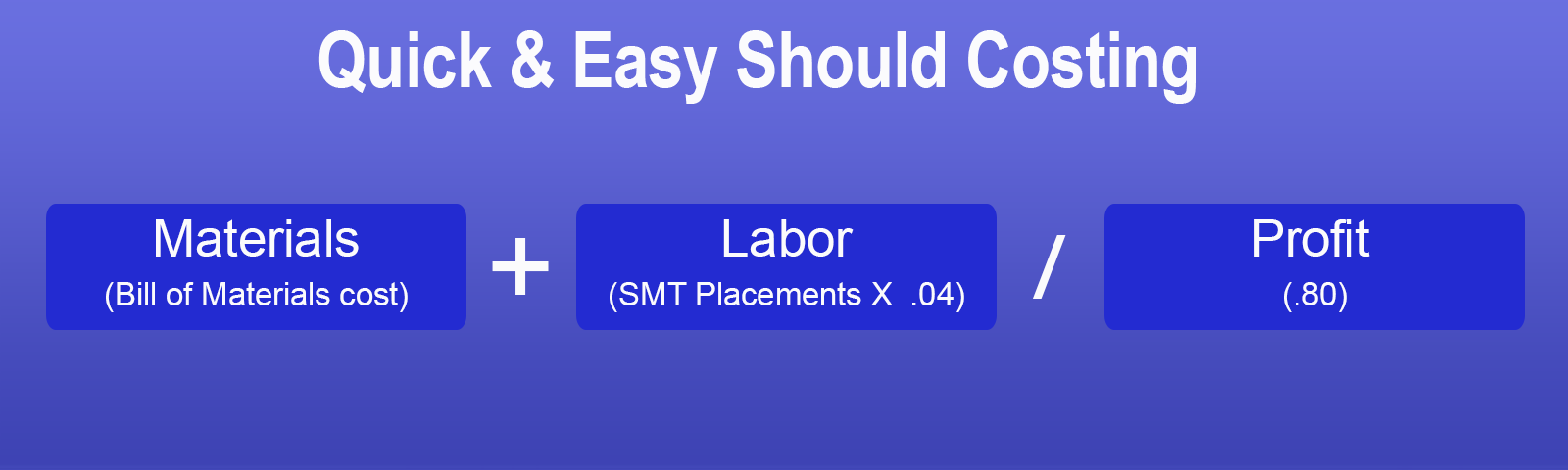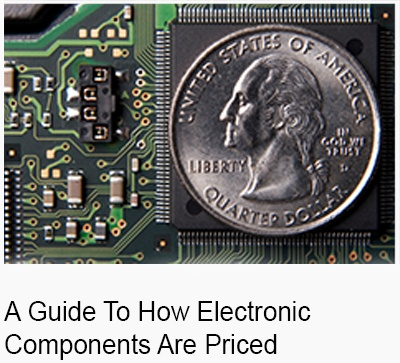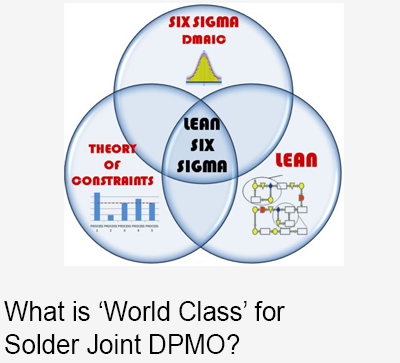Conducting a should cost analysis can seem daunting but is actually very achievable for most OEM's outsourcing electronic assemblies. Use this quick and easy guide to benchmark your current price for an individual PCB assembly and determine if it is competitive.Step 1: Get your material cost

• If you already know it, go to step 2, or else...
• Get it from your PCB assembly supplier. Ask them for the total BOM cost, including PCB (bare) fab. They will give you the total BOM cost with (relatively) little resistance. They won't like being asked for line by line costs, but you don't need that detail for this quick & easy method. If they won't give the total BOM cost to you, be very insistent, and if that still doesn't work...
• Estimate the material yourself. Gather the prices from online distribution sources like Digikey. Use the price column closest to your estimated annual usage. Because online prices are higher than production volume prices, reduce the online prices by 20% to estimate total BOM cost.

Step 2: Estimate labor cost

• Count the number of SMT placements. Do this by summing the "quantity per" column on your BOM.
• Multiply your SMT placements by .10 for mid-volume (less than \$5 million annual). The result is typically between 8-10% of your material cost. You can adjust the .10 multiplier down to as low as .01 if necessary to get labor into this 8-10% zone. If labor is higher than 10% it may be worth getting a more precise labor estimate. If labor is less than 5% of material, don't worry about it.
• Add .05 for each through hole component if there are 1 to 15  through hole components (typically connectors). If there are more than 15 use .03.Step 3: Calculate Profit

• A reasonable expectation in this model is 20% gross profit.
• To calculate 20% gross profit, divide the sum of costs by 1 -Profit Margin. So if you want to calculate a resale based on 20% profit margin, divide the cost by 1 - .20 = .80.
• You may think 20% is too high, it's probably not. EMS companies manipulate the labor, overhead, and profit to show customers what they want to see.

Example

• Materials \$900, 230 SMT placements, 20% profit margin: (900 + [230 x .04]) / .80 = 1136.50

How to use the results

• If your actual prices paid are within +/- 5% of this model, your likely getting a good price (read why) and don't need to worry about this assembly.
• If your actual prices are higher than the model by 5% to 15%, there is a reasonable chance you can lower your prices by 5-10%. Calculate how much this means in real dollars and decide if it is worth pursuing.
• If your price is above the model by more than 15%, you have an excellent opportunity to save 10% or more.

## SUBSCRIBE NOW

### KEEP READING